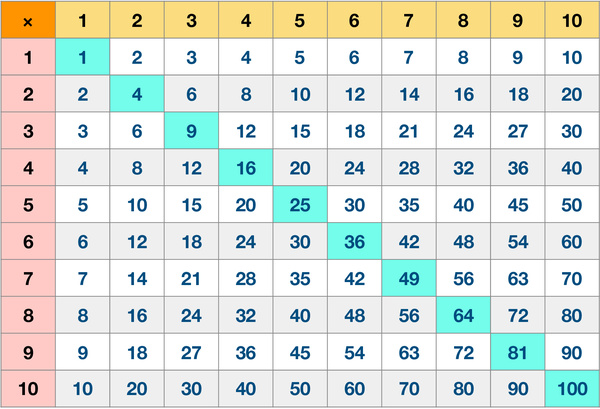# Multiplication Table 10 by 10In mathematics, a multiplication table or, a times table, is a mathematical table used to define a multiplication operation for numbers. The multiplication table was traditionally taught as an essential part of elementary arithmetic around the world, as it lays the foundation for arithmetic operations with base-ten numbers. Memorizing the multiplication table is an important skill for learning mathematics. Many educators believe it is necessary to memorize the table up to 10 × 10. Once kids master this skill, they could do calculations quickly and accurately without using calculators.

This worksheet contains a chart of multiplication results of factors from 1 to 10. It is better to introduce the multiplication table to kids as soon as they start learning numbers and counting. With the help of this chart, parents could help kids learn, memorize, practice and recall multiplications of small numbers easily.